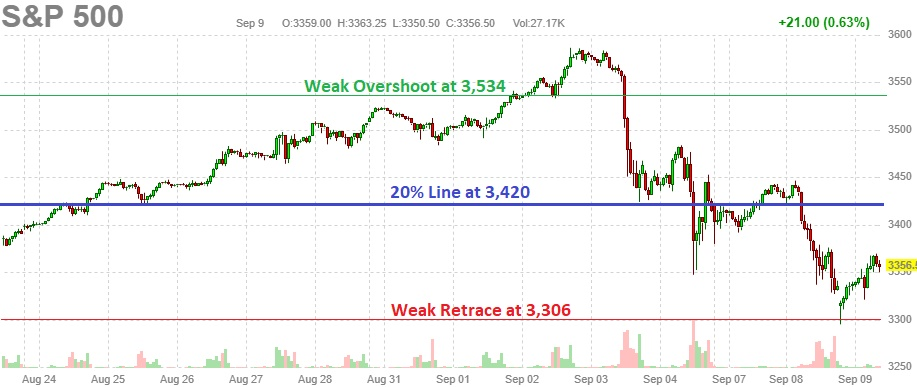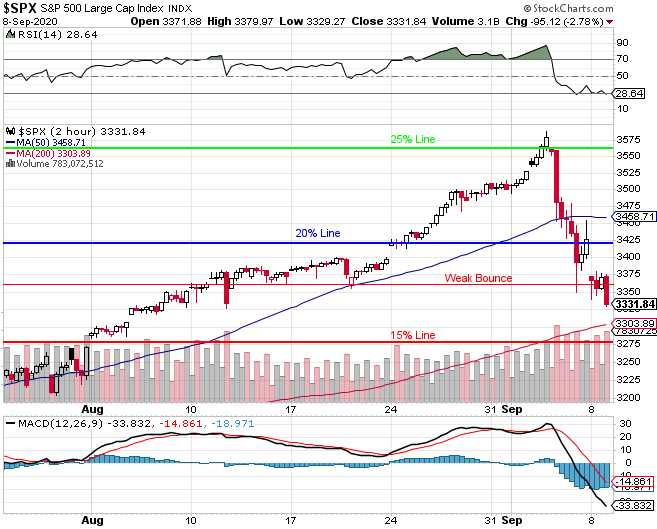# Which Way Wednesday – More Stimulus EditionWell, that was predictable.

How predictable?  So predictable that I wrote on Sunday:

Remember, the 5% Rule™ is not TA, TA is nonsense, the 5% Rule™ is MATH!  Math is how we describe the universe – including the market universe.  The math of the 5% Rule™ tells us that 25% (3,562) is just the 20% line (3,420) with an overshoot but now the key is how much of a pullback do we get?  If the 20% line holds, then this could be bullish consolidation for a move up but, if it fails, then we can expect at least a 20% retrace of the 20% move up (-4%) and that would be 3,420-2,850 = 570 x 0.2 = 114.  So 3,420 – 114 = 3,306 – that's the pullback line we need to keep an eye on.

As usual, the bounce is more predictable than the recovery but the bottom line is both parties are, once again, talking about stimulus because they know as well as I do that failing to hold 3,306 would take us down to the strong retrace line at 3,135 and that would be more than a 10% correction off the 3,588 high (3,229), which would set off all kinds of panicky indicators so GAME ON for more stimulus talks – even if it is the same BS they were arguing over in June and July with no resolution (they took August off to watch the country burn).

So of course we're going to bounce off the line we predicted we'd bounce off.  The question is – how much?  Here's where the 5% Rule™ gets a little tricky because there's two zones we're looking at.  One is easy, that's simply the total drop from 3,600 (we give them the extra 12 points) to 3,300 and that's 300 points so we expect 60-point bounces to 3,360 (weak) and 3,420 (strong) and, since 3,420 is our 20% line – that's going to be a very serious win/lose line for the S&P 500.The other calculation we can do is going to be more accurate and that's using our 5% lines from 2,850, which is the Must Hold line on the S&P 500 (the line below which we are no longer in…